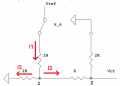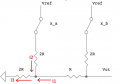# Deciding Vout, switches/digital

#### beggi9

Joined Mar 3, 2016
42
I'm not really sure where to begin here, this is our first problem regarding these kinds of circuits.
I need to determine Vout for the following circuit (see attachment) when the voltage for the switches are Vref*xa and Vref*xb or 0. My best guess would be that if both switches are to the ground then Vout would be zero. In the other cases I'm more unsure, if the switch to the right (x_b) would be connected to the ground and the other switch to Vref, then I think Vout would also be zero. In the other cases I'm really not sure.

Any help well appreciated!

#### WBahn

Joined Mar 31, 2012
28,480
Redraw the circuit as a somewhat complex voltage divider where Vref is at the top and ground is at the bottom and the various resistors are placed between the two accordingly for the state of the switches (which means that the redrawn circuit has no switches, just resistors between Vref and ground). Then it should become apparent what the voltage at the output is (or at least how to find it).

#### beggi9

Joined Mar 3, 2016
42
Redraw the circuit as a somewhat complex voltage divider where Vref is at the top and ground is at the bottom and the various resistors are placed between the two accordingly for the state of the switches (which means that the redrawn circuit has no switches, just resistors between Vref and ground). Then it should become apparent what the voltage at the output is (or at least how to find it).
Okay so when x_a is open and x_b to the ground I get Vout= 1/3*Vref*(x_a) (I look at it like two voltage dividers)
(2R/(2R+2R)) * Vref * (x_a) = 1/2*Vref*(x_a) then (1/2*Vref*(x_a))*((2R)/(3R))=1/3*Vref*(x_a)

When x_b is open and x_a is to the ground I get Vout = ((R+(1/R))/((3R)+(1/R)))*Vref*(x_b)

I'm still not seeing how I would go about solving it when both of them are open (have Vref)

#### WBahn

Joined Mar 31, 2012
28,480
Draw the circuit and post it. Then describe what the currents in the resistors must be and what that means about the output voltage.

#### beggi9

Joined Mar 3, 2016
42
Draw the circuit and post it. Then describe what the currents in the resistors must be and what that means about the output voltage.
This is what I was thinking for when x_a is open and x_b is to the ground
But yeah it says in the problem to look at the currents and that no current flows to Vout

#### beggi9

Joined Mar 3, 2016
42
Here is the drawing of when x_a is connected to the ground and x_b is open

#### WBahn

Joined Mar 31, 2012
28,480
This is what I was thinking for when x_a is open and x_b is to the ground
But yeah it says in the problem to look at the currents and that no current flows to Vout
You have a note in red that says that the current splits 50-50. What current? Splits where? Why 50-50?

#### WBahn

Joined Mar 31, 2012
28,480
Here is the drawing of when x_a is connected to the ground and x_b is open
Look at the units in your expression (in the attached drawing). Do they work out?

Consider just the denominator. You have 2R + R + (1/R).

How can you add R to (1/R)? What would the units be?

•beggi9

#### beggi9

Joined Mar 3, 2016
42
Look at the units in your expression (in the attached drawing). Do they work out?

Consider just the denominator. You have 2R + R + (1/R).

How can you add R to (1/R)? What would the units be?
Yes sorry that should be R not 1/R and would give Vout=1/2*Vref*(x_b)

You have a note in red that says that the current splits 50-50. What current? Splits where? Why 50-50?
I might be mistaken but I thought at point 1 there would be a voltage divider where the voltage for the right side would be
Vright = "(2R/4R)*Vref*(x_a) (or half of the original Vin)

#### WBahn

Joined Mar 31, 2012
28,480
How are you getting (2R/4R)?

We can't spot where you are making your mistake when all you give is a final result.

Take it step by step.

#### beggi9

Joined Mar 3, 2016
42
How are you getting (2R/4R)?

We can't spot where you are making your mistake when all you give is a final result.

Take it step by step.
Okay I'm sorry, I thought that at point 1 (in picture 1) there would be a voltage divider. I use that to find what voltage on the right side would be (that is Vright). Because we have two resistances of 2R I concluded that Vrigh would be:
Vright = (2R/(2R+2R))*Vin (here Vin would be Vref*(x_a) as mentioned in the beginning)
= (2R/4R)*Vref*(x_a)
= 1/2*Vref*(x_a)
Now knowing the voltage at the other intersection I would again look at it like a voltage divider where Vin = Vright = 1/2*Vref*(x_a).
So Vout = (R/2R+R)*Vin
= 1/3*1/2*Vref*(x_a)=1/6*Vref*(x_a)

#### WBahn

Joined Mar 31, 2012
28,480
I don't know what you mean by "the voltage on the right side". Do you mean the voltage at node 2? Also known as Vut?The only place that current can split is at Node 1.

Current comes down (I1) and then splits into I2 and I3. Your note says that this splits 50-50. Does that make sense?

How much resistance is there from Node 1 to ground via the path followed by I2?

How much resistance is there from Node 1 to ground via the path followed by I3?

If these aren't the same but if I2 = I3, then you will get different voltages at Node 1 depending on which path you follow.

•beggi9

#### beggi9

Joined Mar 3, 2016
42
I don't know what you mean by "the voltage on the right side". Do you mean the voltage at node 2? Also known as Vut?

The only place that current can split is at Node 1.

Current comes down (I1) and then splits into I2 and I3. Your note says that this splits 50-50. Does that make sense?

How much resistance is there from Node 1 to ground via the path followed by I2?

How much resistance is there from Node 1 to ground via the path followed by I3?

If these aren't the same but if I2 = I3, then you will get different voltages at Node 1 depending on which path you follow.
No I does not make sense for the I2=I3 (that is 50/50 split). The resistance from Node 1 to ground via the path followed by I2 is 3R and the resistance from Node 2 to ground via the path followed by I3 is 2R.
What I meant was that the voltage drop would be even over the 2R (via I3) and over the R+2R (via I2).
But okay I can see my error. So looking at the currents I get:
I1=I2+I3 ----- (x_a)*Vref = I1*2R + 2R*I3 ------- (x_a)*Vref=I2*3R + I1*2R
so we have 2R*I3=I2*3R ----> I3=(3/2)*I2
(x_a)*Vref = 2.5*I2*2R+I2*3R=8*I2*R
now Vut=(x_a)*Vref-I2*2R=6*I2*R
there for Vut = (6/8)*(x_a)*Vref= (3/4)*(x_a)*Vref
Or am I mistaken?

#### beggi9

Joined Mar 3, 2016
42
And I could use the same principle when (x_b) is to Vref and (x_a) to the ground.
Then I would have I1=I2+I3
Vref*(x_b)=(3R)*I1+2R*I2
Vref*(x_a)=(3R)*I1+2R*I3
so I2=I3
Vref*(x_b)=3R*2*I2+R*2*I2=8*I2*R
Vut = Vref*(x_b)-2*I2*2R=4*I2*R
Vut = (1/2)*Vref*(x_b)

But I'm still very uncertain how it would go when both are open to Vref

#### WBahn

Joined Mar 31, 2012
28,480
You keep talking about switches being "open". But I see switches that are NEVER open, they either tie the common terminal to Vref or they tie the common terminal to Ground. In either case, current can flow through the switch, which would not be the case if it were "open".

•beggi9

#### beggi9

Joined Mar 3, 2016
42
You keep talking about switches being "open". But I see switches that are NEVER open, they either tie the common terminal to Vref or they tie the common terminal to Ground. In either case, current can flow through the switch, which would not be the case if it were "open".
Sorry I mean when both switches are connected to Vref.

#### WBahn

Joined Mar 31, 2012
28,480
Sorry I mean when both switches are connected to Vref.
Okay, so connect both circuits to Vref.

There are multiple ways to solve it from there. Perhaps conceptually the "simplest" is to figure out the total resistance from Vref to ground and find the total current flowing in the circuit. Then use that information to find the voltage at Node 1. Then use that to find the current flowing down the branch containing Vut. Then use that to find the voltage at Vut.

•beggi9

#### beggi9

Joined Mar 3, 2016
42
Okay, so connect both circuits to Vref.

There are multiple ways to solve it from there. Perhaps conceptually the "simplest" is to figure out the total resistance from Vref to ground and find the total current flowing in the circuit. Then use that information to find the voltage at Node 1. Then use that to find the current flowing down the branch containing Vut. Then use that to find the voltage at Vut.
hmmm okay, this is my attempt at solving it:We have Vref*(x_b)=I1*(3R)+I3*2R
Vref*(x_a)=I2*(2R)+I3*2R
I3=I2+I1
I then assumed that Vref*(x_b)=Vref*(x_a) and got
I2=(3/2)*I1
So Vut = (3/4)*Vref*(x_b)
I now however suspect that this assumption would be wrong and that I should try so solve it involving both Vref*(x_a) and Vref*(x_b)?

#### WBahn

Joined Mar 31, 2012
28,480
In all of these equations, are (x_a) and (x_b) Boolean values (i.e., either a 0 or a 1)?